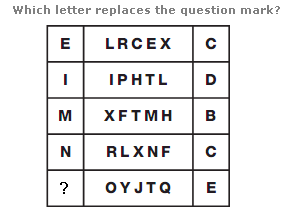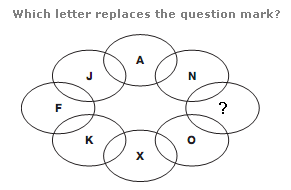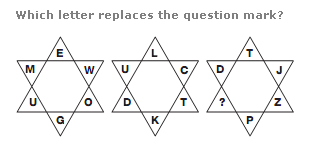# Puzzles - Missing letters puzzles

### Exercise :: Missing letters puzzlesAnswer : Q Explanation : Converting all letters to their numerical values, the middle section of each row of the diagram contains a list of letters which are common multiples of a number, given by the value of the letter in the right hand box. One letter in each row does not follow this sequence, and is put in the left hand box.Answer : S Explanation : Join pairs of letters at opposite sides of the diagram, with lines which run through the centre. The sum of the numerical values of the pairs of letters is always 25.Answer : N Explanation : In each star, start at the bottom and move anti-clockwise around the points. Letters follow the alphabetic sequence, in steps of 8 letters, for the left hand circle, 9 for the central one and 10 for the right hand circle.